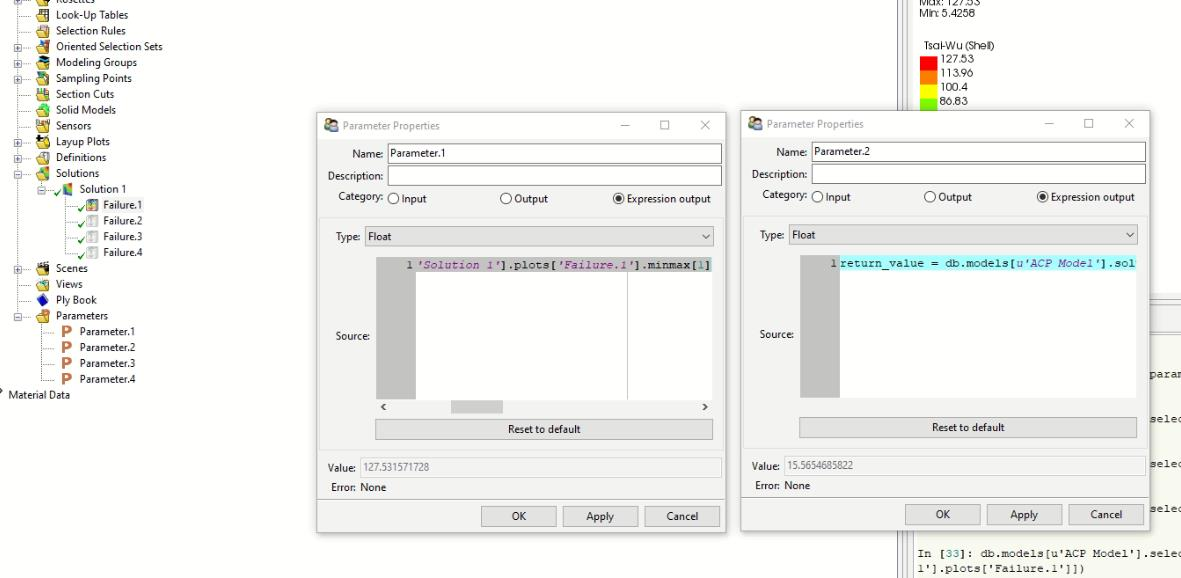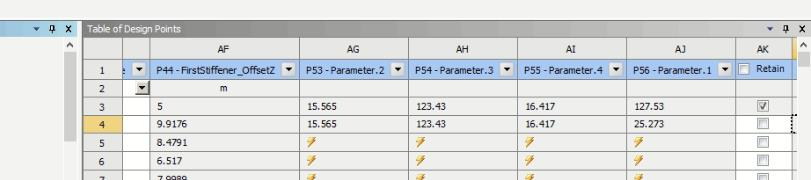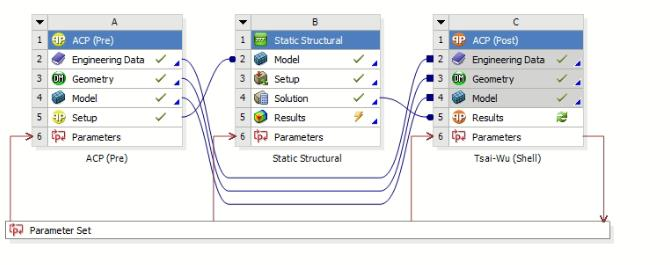# ACP parameter set problem

Hi, everyone!

I’m using ACP to analyze some structures, but I got a problem with the parameter set.

When I add the parameters in ACP(Post). For example, I tried four different failure criteria results.

The code for four parameters I put are:

return_value = db.models[u'ACP Model'].solutions['Solution 1'].plots['Failure.1'].minmax

return_value = db.models[u'ACP Model'].solutions['Solution 1'].plots['Failure.2'].minmax

return_value = db.models[u'ACP Model'].solutions['Solution 1'].plots['Failure.3'].minmax

return_value = db.models[u'ACP Model'].solutions['Solution 1'].plots['Failure.4'].minmaxAs you can see the value have shown the value I want from the solution, but when I went to the parameter set, the first DoE will record these four values, However, the following DoE only have one Parameter.1 updated! The rest three parameters didn’t update as I want and only copy the first DoE results.

What should I do to have all the values updated? Or something wrong with the code I put or the link between blocks?

Regards

Wenxin

•